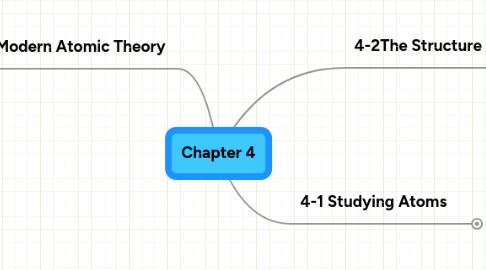# Chapter 4

Get Started. It's FreeChapter 4## 1. 4-1 Studying Atoms

### 1.1. Dalton's theory

1.1.1. All elements are composted of atoms

1.1.2. All atoms of the same element have the same mass, and atoms of different elements have different masses.

1.1.3. Compounds contain atoms of more than one element.

1.1.4. In a particular compound, atoms of different elements always combine in the same way.

1.1.5. Elements are pictured as solid spheres

### 1.2. Thomson's Model

1.2.1. Thomson preformed experiments gave the first evidence that atoms are made of even smaller particles

1.2.2. Thomson concluded that an atom is neutral, meaning it had neither a negative or positive charge.

1.2.3. In Thomson's model the negative charges were evenly scattered throughout an atom filled with positively charged particles. Also known as the "plum pudding" model.

### 1.3. Rutherford's Atomic Theory

1.3.1. Discovered uranium emits fast-moving particles that have a positive charge, he named the alpha particles.

1.3.2. Gold Foil Experiment

1.3.2.1. Marsden (Rutherford's student) aimed a narrow beam of alpha particles at a gold sheet, and expected all of the particles to pass though the gold.

1.3.2.2. 1/20,000 of the particles was deflected by more than 90º, some even bounced straight back.

1.3.3. Discovery of the Nucleus

1.3.3.1. Rutherford concluded from the experiment that the positive charge of an atom is not even spread throughout the atom. TI is concentrated in a small central area called the nucleus.

1.3.3.2. According to Rutherford's model, all of an atom's positive charge is concentrated in its nucleus.

## 2. 4-3 Modern Atomic Theory

### 2.1. Bohr Model

2.1.1. Energy levels

2.1.1.1. The possible energies that electrons in an atom can have are called energy levels

2.1.1.2. Electrons cannot exist between energy levels.

2.1.1.3. An electron in an atom can move from one energy level to another when the atom gains of loses energy.

### 2.2. Lewis Dot Structure

2.2.1. Diagrams that show bonding between atoms of a molecule, and the lone pairs of electrons that may exist in the molecule

2.2.2. New node

### 2.3. Electron Could Model

2.3.1. An electron could is a visual model of the most likely locations for electrons in an atom.

2.3.2. The could is denser at those locations where the probability of finding an electron is high.

2.3.3. Scientists use the electron could model to describe the possible locations of electrons around the nucleus.

### 2.4. Atomic Orbitals

2.4.1. An orbital is a reighon of space around the nucleus where an electron is likely to be found.

2.4.2. An electron could is a good approximation of how electrons behave in their orbitals.

### 2.5. Electron Configurations

2.5.1. An electron configuration is the arrangement of electrons in the orbitals of an atom.

2.5.2. The most stable electron configuration is the one in which the electrons are in orbitals with the lowest possible energies.

2.5.3. When all the electrons in an atom have the lowest possible energies, the atom is said to be in its ground state

## 3. 4-2The Structure of an Atom

### 3.1. Properties of Subatomic Particles

3.1.1. Protons

3.1.1.1. A proton is a positively charged subatomic particle that is found in the nucleus of an atom.

3.1.1.2. Each proton is assigned a charge of 1 +

3.1.2. Electrons

3.1.2.1. An electron is a negatively charged subatomic particle taht is found in the space outside the nucleus.

3.1.2.2. Each electron has a charge of 1 -

3.1.3. Neutrons

3.1.3.1. A neutron is a neutral subatomic particle that is found in the nucleus of an atom.

3.1.3.2. A neutron has a mass almost exactly equal to that of a proton

### 3.2. Comparing Subatomic Particles

3.2.1. Protons, electrons, and neutrons can be distinguished by mass, charge, and location in an atom.

3.2.2. Protons and neutrons have almost the same mass, but it would take about 2,000 electrons to equal the mass of one proton.

3.2.3. Electrons have a charge that is equal in saize to, but the opposite of, the charge of a proton.

3.2.4. Protons and neutrons are found in the nucleus, but electrons are found in the space outside the nucleus

### 3.3. Atomic Number and Mass Number

3.3.1. Atomic Number

3.3.1.1. The atomic number of an element equals the number of protons in an atom of that element.

3.3.1.2. Atoms of different elements have different numbers of protons.

3.3.1.3. Each positive charge in an atom is balanced by a negative charge because atoms are neutral, so the atomic number of an element also equals the number of electrons in an atom.

3.3.2. Mass Number

3.3.2.1. The mass number of an atom is te sum of the protons and neutrons in the nucleus of that atom.

### 3.4. Isotopes

3.4.1. Isotopes are atoms of the same element that have different number of neutrons and different mass numbers.

3.4.2. Isotopes of an element have the same atomic number but different mass number because they have different numbers of neutrons.